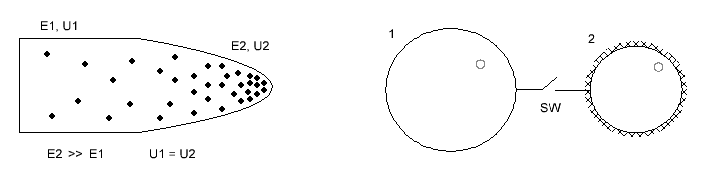Research website of Vyacheslav Gorchilin
2016-08-11
About the relativity of the potential energy of the capacitor
At the junction of two branches of physics — electrostatics and electrodynamics — there are a number of little studied effects. Although at first glance are not obvious, they are fully explained by the classical models. One of these effects we consider in this article.
1. Some properties of the surface of the plates of the capacitor
We will start with the school experience , where the operator is using the electroscope examines the charge density on the combined surface of the cylinder with the cone. First, the operator removes the charge from the cone then the cylinder. The result is that the cone had more (left figure). Thus, the charge distribution on the conductor surface depends on its curvature: where it more there more rushes of charges. Now if somehow instantly split the cone and cylinder, then the cone will have more charges. This effect we will consider in future.
An interesting question arises with the potential. From the General formula it follows that to obtain it you need to bypass the whole length: $\Delta\varphi = \int \limits _{L} {\vec E} \, d {\vec \ell} \qquad (1),$ but since we share the surface, it is obvious that we need to talk about some local or local potential. This will complicate the understanding of the effect, so we will not use, and will reflect all processes using a charge and capacity.The figure on the right shows the two balls with different radii: 1-St — more 2nd. The surface of the second ball covered with needle grid, such that its pointed end facing away from the center, perpendicular to the surface. First charge of the 1st ball, then we close the switch SW. On the basis of the first experiment, we can assume that despite the smaller diameter, most of the charge will move to the second ball. The ratio of the transition will depend on the architecture of the second ball. If you now open the switch SW, the charge on the ground will be less than on the second.
Now we come to the most interesting expression of the process using the ratio of the energies. To do this, first we find the energy spent on charging 1-ball: $W_0 = { Q^2 \over 2\,C_1 } \qquad (2)$ Then we present the potential of energy all the way two balls after opening key: $W_1 = { Q_1^2 \over 2\,C_1 }, \, W_2 = { Q_2^2 \over 2\,C_2 } \qquad (3)$ where $$Q_1$$ is the charge first ball, $$Q_2$$ — charge the second balloon. In compliance with the law of conservation of charge it is clear that $$Q = Q_1 + Q_2$$. Also, we need a coefficient that shows the ratio of the transition charge: $g = { Q_2 \, C_1 \over Q_1 \, C_2 } \qquad (4)$ When surfaces of the two capacitors are the same, this coefficient is equal to one. School experience  and it is intuitively clear that for $$g \gt 1$$ the surface of the first capacitor needs to be smooth, and the second on the contrary — the more uneven, then the charges will actively strives for it to go. In fact $$g$$ is the ratio of the geometry of surfaces. Note that the analytically deduced it quite difficult, much easier to obtain experimentally. The same factor may explain the apparent discrepancy of the effect Biffeld–brown with the classical model .
On this basis we calculate the elapsed and the received energy is: $K = { W_1 + W_2 \over W_0 } = { 1 + g^2 {C_2 \over C_1} \over \left( 1 + g {C_2 \over C_1} \right)^2 } \qquad (5)$ As you can see, if $$g = 1$$, then $$K$$ will always be less than unity. Our task — to increase $$g$$ by changing the architecture of the surface of the second capacitor. If it is much greater than unity, the formula (4) are greatly simplified: $K \approx {C_1 \over \ C_2}, \, g \gg 1 \qquad (6)$ the Obtained energy increment is the increase coefficient of efficiency of the second kind: $K = K_{\eta2}$ Next, we show how the obtained results apply in practice.